Courses

# NEET Previous Year Questions (2014-20): Current Electricity Class 12 Notes | EduRev

## NEET : NEET Previous Year Questions (2014-20): Current Electricity Class 12 Notes | EduRev

The document NEET Previous Year Questions (2014-20): Current Electricity Class 12 Notes | EduRev is a part of the NEET Course Physics 31 Years NEET Chapterwise Solved Papers.
All you need of NEET at this link: NEET

Q.1. Which of the following graph represents the variation of resistivity (r) with temperature (T) For copper?       (2020)
A: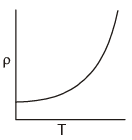B: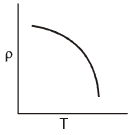C: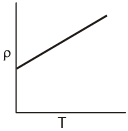D: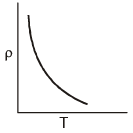Ans: A
Relation between resistivity and temperature is given by ρ = ρ0e-αT

Q.2. A charged particle having drift velocity of 7.5×10-4 m s-1 in an electric field of 3×10-10 Vm-1 has a mobility in m2 V-1 s-1 of :        (2020)
A: 2.5×10-6
B: 2.25×10-15
C: 2.25×1015
D: 2.5×106
Ans:
D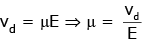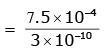μ = 2.5 × 106
(μ = mobility)

Q.3. The color code of a resistance is given below: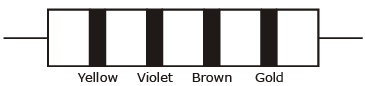The values of resistance and tolerance, respectively, are :        (2020)
A: 4.7 kΩ, 5%
B: 470Ω, 5%
C: 470 kΩ, 5%
D: 47 kΩ, 10%

Ans: B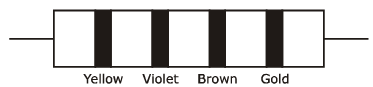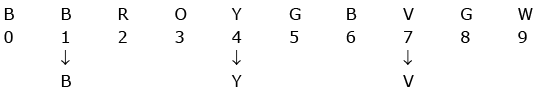R = 47 × 101Ω ± 5%
= 470 Ω ± 5%
So, 470, 5%

Q.4. The solids which have the negative temperature coefficient of resistance are :        (2020)
A: semiconductors only
B: insulators and semiconductors
C: metals
D: insulators only

Ans: B
Insulators have a negative temperature coefficient because as temperature increases, the resistance of insulators decreases. The resistivity of the semiconductor material to decrease with the rise in temperature, resulting in a negative temperature coefficient of resistance.

Q.5. Six similar bulbs are connected as shown in the figure with a DC source of emf E and zero internal resistance.        (2019)
The ratio of power consumption by the bulbs when (i) all are glowing and (ii) in the situation when two from section A and one from section B are glowing, will be :      (2019)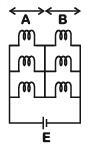A: 4 : 9
B: 9 : 4
C: 1 : 2
D: 2 : 1
Ans:
B
Solution:

(i) All bulbs are glowing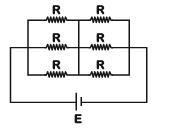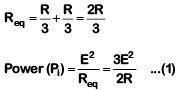(ii) Two from section A and one from section B are glowing.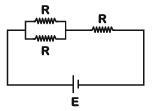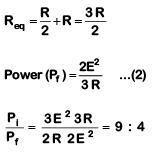Q.6. In the circuits shown below, the readings of the voltmeters and the ammeters will be      (2019)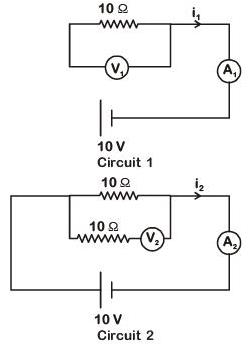A: V2 > V1 and i1 = i2
B: V1 = V2 and i1 > i2
C: V1 = V2 and i1 = i2
D: V2 > V1 and i1 > i2

Ans: C
Solution:
For ideal voltmeter, resistance is infinite and for the ideal ammeter, resistance is zero.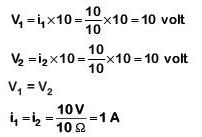Q.7. A set of 'n' equal resistors, of value 'R' each, are connected in series to a battery of emf 'E' and internal resistance 'R'. The current drawn is I. Now,the 'n' resistors are connected in parallel to the same battery. Then the current drawn from battery becomes 10 I. The value of 'n' is :-    (2018)
A: 10
B: 11
C: 20
D: 9
Ans:
A
Solution: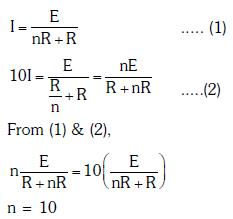Q.8. A battery consists of a variable number 'n' of identical cells (having internal resistance 'r' each) which are connected in series. The terminals of the battery are short-circuited and the current I is measured. Which of the graphs shows the correct relationship between I and n ?   (2018)
A: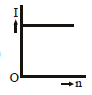B: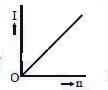C: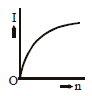D: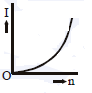Ans: A
Solution: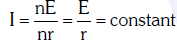Q.9. Current sensitivity of a moving coil galvanometer is 5 div/mA and its voltage sensitivity (angular deflection per unit voltage applied) is 20 div/V. The resistance of the galvanometer is     (2018)
A: 40 Ω
B: 25 Ω
C: 250 Ω
D: 500 Ω
Ans: C
Solution: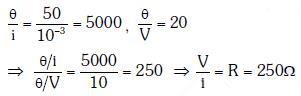Q.10. The resistance of a wire is 'R' ohm. If it is melted and stretched to 'n' times its original length, its new resistance will be :    (2017)
A: R/n
B: n2R
C: R/n2
D: nR
Ans: B
Solution: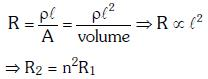Q.11. A potentiometer  is an accurate and versatile device to make electrical measurements of E.M.F. because the method involves :-     (2017)
B: A condition of no current flow through the galvanometer
C: A combination of cells, galvanometer and resistances
D: Cells
Ans:
B
Solution:

In zero deflection condition, potentiometer draws no current.

Q.12. A potentiometer wire is 100 cm long and a constant potential difference is maintained across it. Two cells are connected in series first to support one another and then in opposite direction. The balance points are obtained at 50 cm and 10 cm from the positive end of the wire in the two cases. The ratio of emf's is :      (2016)
A: 3:2
B: 5:1
C: 5:4
D: 3:4
Ans:
A
Solution:
According to question, emf of the cell is directly proportional to the balancing length.
i.e., E∝ ℓ    ... (i)
Now, in the first case, cells are connected in series
That is,
Net EMF = E1 + E2
From equation (i),
E1 + E2 = 50 cm (given)  ... (ii)
Now, the cells are connected in series in the opposite direction,
Net emf = E1 - E2
From equation (i)
E1 - E2 = 10       ... (iii)
From equation (ii) and (iii),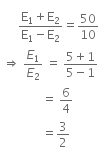Q.13. The charge flowing through a resistance R varies with time t as Q = at - bt2, where a and b are positive constants. The total heat produced in R is :     (2016)
A: a3R/b
B: a3R/6b
C: a3R/3b
D: a3R/2b
Ans: B
Solution:

Given,
Charge, Q = at - bt2   ... (i)
We know that,
Current, I = dθ/dt
So, equation (i) can be written as,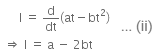For maximum value of t, the current is given by,
a-2bt = 0
Therefore, t = a/2b    ..(iii)
Total heat produced (H) can be given as,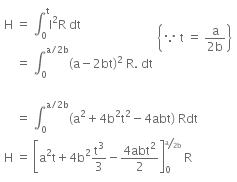On solving the above equation, we get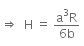Given,
Charge, Q = at - bt2   ... (i)
We know that,
Current, I = dθ/dt
So, equation (i) can be written as,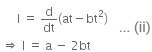For maximum value of t, the current is given by,
a-2bt = 0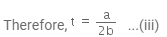Total heat produced (H) can be given as,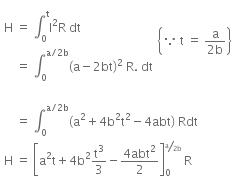On solving the above equation, we get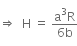Q.14. A, B and C are voltmeters of resistance R, 1.5 R and 3R respectively as shown in the figure. When some potential difference is applied between X and Y, the voltmeter readings are VA, VB and VC respectively.     (2015)
Then :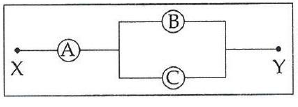A: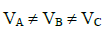B: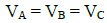C: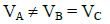D: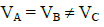Ans: B
Solution: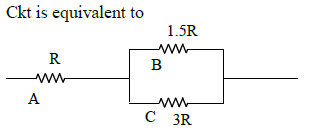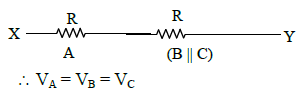Q.15. A potentiometer wire has length 4 m and resistance 8 Ω. The resistance that must be connected in series with the wire and an accumulator of e.m.f. 2V, so as to get a potential gradient 1 mV per cm on the wire is :     (2015)
A: 48 Ω
B: 32 Ω
C: 40 Ω
D: 44 Ω
Ans:
B
Solution: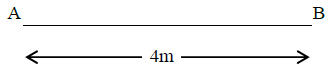Figure alongside shows a potentiometer wire of length L = 4m and resistance RAB = 8Ω. Resistance connected in series is R.
When an accumulator of emf ε = 2V is used, we have current I given by,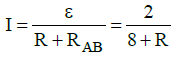The resistance per unit length of the potentiometer wire is given by,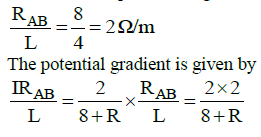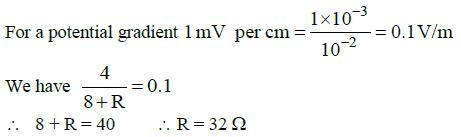Q.16. Across a metallic conductor of non-uniform cross section a constant potential difference is applied. The quantity which remains constant along the conductor is :  (2015)
A: electric filed
B: current density
C: current
D: drift velocity
Ans:
C
Solution:
As the cross-sectional area of the conductor is non-uniform so current density will be different.
As, I = JA
It is clear from Eq. (i) when an area increases the current density decreases so the number of the flow of electrons will be same and thus the current will be constant.

Q.17. In an ammeter 0.2% of main current passes through the galvanometer. If resistance of galvanometer is G, the resistance of ammeter will be:     (2014)
A: 1/500 G
B: 500/499 G
C: 1/499 G
D: 499/500 G
Ans:
A
Solution:

Let the shunt resistance be S.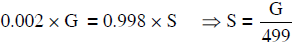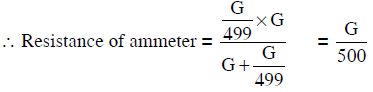Q.18. The resistance in the two arms of the meter bridge are 5 Ω and R Ω, respectively. When the resistance R is shunted with an equal resistance, the new balance point is at 1.6ℓ1. The resistance ‘R’ is :    (2014)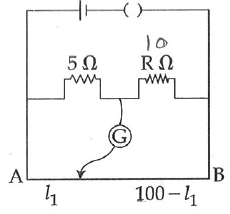A: 20 Ω
B: 25 Ω
C: 10 Ω
D: 15 Ω
Ans: B
Solution: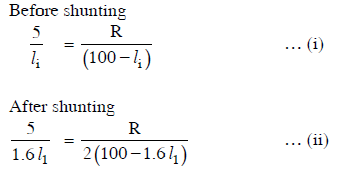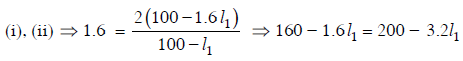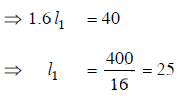Q.19. Two cities are 150 km apart. Electric power is sent from one city to another city through copper wires. The fall of potential per km is 8 volt and the average resistance per km is 0.5 Ω. The power loss in the wire is :    (2014)
A: 19.2 J
B: 12.2 kW
C: 19.2 W
D: 19.2 kW
Ans:
D
Solution:
Total voltage drop across wire = 150 x 8
= 1200 volt
Total resistance of wire
=  150 x 0.5 = 75 Ω
∴ current through wire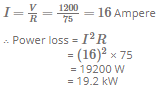Q.20. A potentiometer circuit has been set up for finding the internal resistance of a given cell. The main battery, used across the potentiometer wire, has an emf of 2.0 V and a negligible internal resistance. The potentiometer wire itself is 4 m long. When the resistance, R connected across the given cell, has value of.
(i) infinity
(ii) 9.5 Ω, the ‘balancing lengths’ , on the potentiometer wire are found to be 3m and 2.85m,respectively.
The value of internal resistance of the cell is:    (2014)
A: 0.5 Ω
B: 0.75 Ω
C: 0.25 Ω
D: 0.95 Ω
Ans:
A
Solution: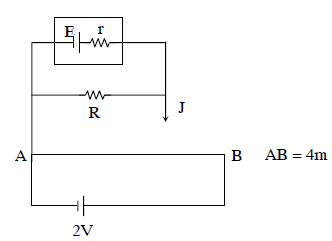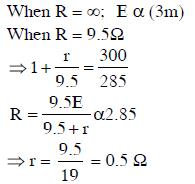Offer running on EduRev: Apply code STAYHOME200 to get INR 200 off on our premium plan EduRev Infinity!

28 docs|50 tests

,

,

,

,

,

,

,

,

,

,

,

,

,

,

,

,

,

,

,

,

,

;